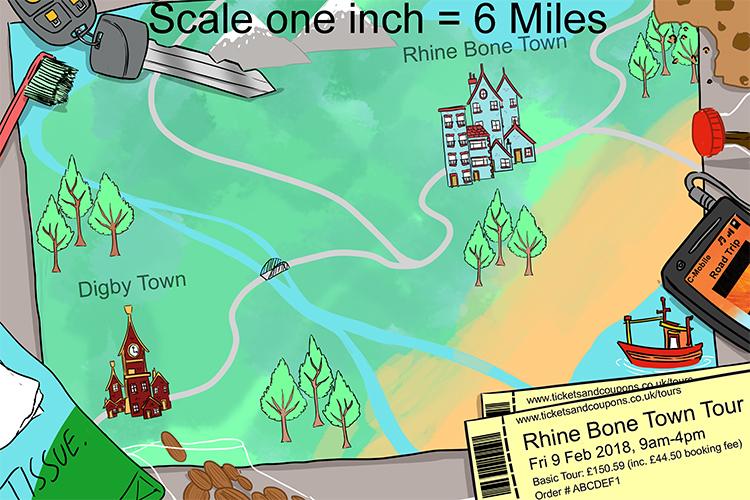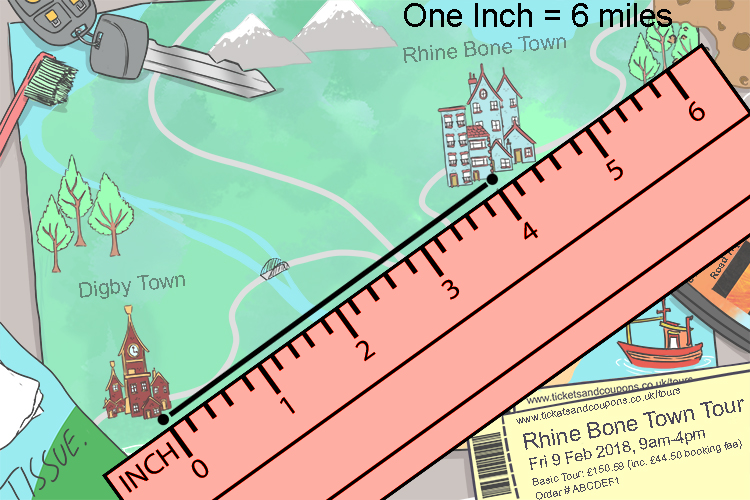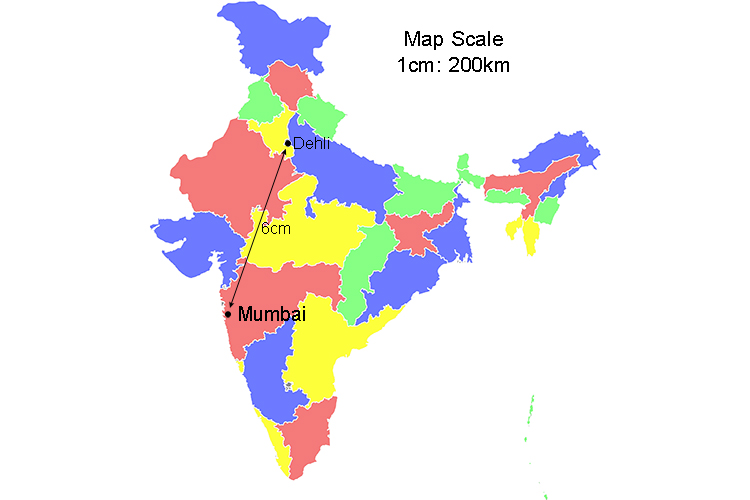# Ratios and maps

To further help you remember what ratios are, remember that the most used ratios in the world are maps.

i.e. The scale of a map is a ratio.

To help you remember ratios is associated with maps (and therefore scale) think of the two people raising their toes (ratio) to the holiday they are going to have (see map on the wall).They raised a toe to everyone (ratio is to every)

because they were going on holiday. Do you see the map?

Example 1

The ratio on a map says that the scale is 1:40,000

So if a measurement of 3.5cm between two points is made what is the actual distance?

1:40,000

is 1 to every 40,000

so 1cm to every 40,000cm

3.5cm on the map =3.5times40,000cm

3.5cm on the map =140,000cm

To make this easier we know that 1metre=1000cm and therefore 140,000cm=140metres

3.5cm on the map =140metres

Example 2

Given the map below how far is it from Digby town to Rhine Bone town?So do this first measure with a ruler from Digby town to Rhine Bone town.You can see that this is 4 inches

One inch to every 6 miles

4 inches would be 4times6m\i\l\es

4times6m\i\l\es=24m\i\l\es

The distance from Digby town to Rhine Bone town is 24\ m\i\l\es.

Example 3

Find the distance from Delhi to Mumbai in the following map.We are told that the map is a scale of 1cm:200km

therefore 6cm would be 6times200km

6times200km=1,200km

The distance from Delhi to Mumbi is 1,200km